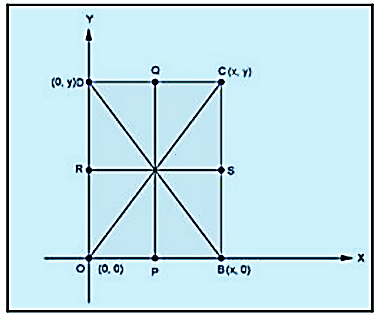# RD Sharma Class 10 Ex 14.4 Solutions Chapter 14 Coordinate Geometry

In this chapter, we provide RD Sharma Class 10 Ex 14.4 Solutions Chapter 14 Coordinate Geometry for English medium students, Which will very helpful for every student in their exams. Students can download the latest RD Sharma Class 10 Ex 14.4 Solutions Chapter 14 Coordinate Geometry pdf, Now you will get step by step solution to each question.

# Chapter 14: Coordinate Geometry Exercise – 14.4

### Question: 1

Find the centroid of the triangle whose vertices are:

(i) (1, 4),( -1, -1) and (3, -2)

### Solution:

We know that the coordinates of the centroid of a triangle whose vertices are

(x1, y1), (x2, y2), (x3, y3) are

So, the coordinates of the centroid of a triangle whose vertices are

(1, 4), (-1, -1) and (3, -2) are

### Question: 2

Two vertices of a triangle are (1, 2), (3, 5) and its centroid is at the origin. Find the coordinates of the third vertex.

### Solution:

Let the coordinates of the third vertex bee (x, y), Then Coordinates of centroid of triangle are

We have centroid is at origin (0, 0)

⇒ x + 4 = 0 ⇒ y + 7= 0

⇒ x = – 4 ⇒ y = – 7

### Question: 3

Prove analytically that the line segment joining the middle points of two sides of a triangle is equal to half of the third side.

### Solution:

Let ABC be a triangle such that BC is along x-axis Coordinates of A, B and C are (x, y), (0, 0) and (x1,y1)

D and E are the mid-points of AB and AC respectively

Coordinates of D are

Coordinates of E are

### Question: 4

Prove that the lines joining the middle points of the opposite sides of a quadrilateral and the join of the middle points of its diagonals meet in a point and bisect one another.

### Solution:

Let OBCD be the quadrilateral P, Q, R, S be the midpoint off OB, CD, OD and BC. Let the coordinates of 0, B, C, D are (0,0),(x, 0), (x, y) and (0,y)Coordinates of P are (x/2, 0)

Coordinates of Q are (x/2, y)

Coordinates of R are (0, y/2)

Coordinates of S are (x, y/2)

Coordinates of midpoint of PQ are

Coordinates of midpoint of RS are

Since, the coordinates of the mid-point of PQ = coordinates of mid-point of RS

∴ PQ and RS bisect each other

### Question: 6

If G be the centroid of a triangle ABC and P be any other point in the plane, prove that

PA2+ PB+ PC= GA+ GB+ GC+ 3GP2

### Solution:

Let A (0, 0), B (a, 0), and C (c, d) are the o-ordinates of triangle ABC

Hence,

i.e.,

let p(x, y)

to Prove:

PA+ PB+ PC= GA2+ GB+ GC+ 3GP2

Or, PA+ PB+ PC= GA2+ GB2+ GC2+ GP2+ GP+ GP2

Or, PA2– GP2+ PB2– GP2+ PC2+ GP= GA+ GB+ GC2

Proof:

PA2 = x+ y2

PB2 = (x – a)2 + y2

P  = (x – c)2 + (y – d)2

L.H.S

Solving R.H.S

GA2+ GB2+ GC2

∴  L. H. S = R. H. S

All Chapter RD Sharma Solutions For Class10 Maths

I think you got complete solutions for this chapter. If You have any queries regarding this chapter, please comment on the below section our subject teacher will answer you. We tried our best to give complete solutions so you got good